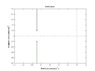# Transfer function to Pole-Zero Plot to Impulse Response Curve

• Bi1a1

## Homework Statement

The question gives a transfer function. and we are asked to put it into pole zero form and then we are asked to sketch the positions of the poles and zeros of the system on the complex plane and then sketch the impulse response corresponding to each pole.

i have uploaded a MATLAB image of my pole-zero plot.
i can do the whole question on MATLAB but that doesn't help with understanding how to solve it.

## Homework Equations

g(s)=1/((s^2)+(18s)+181) ------- Transfer Function

## The Attempt at a Solution

roots of transfer function denominator: ((s^2)+(18s)+181) = (s+(5-8j))(s+(5+8j))

therefore pole zero form:

g(s)=1/((s+(5-8j))(s+(5+8j)))

therefore the diagram of the poles and zeros would have no zeros and two poles at (-5,8) and another at (-5,-8) (Real axis,Imaginary Axis)

i can do upto this part but i am not understanding how to sketch the impulse response curve.

any help would be great.

Thank You.

#### Attachments

•untitled.jpg
10.9 KB · Views: 705
1.What is the transfer function of an impulse?

2. Remember linearity? Let F(s) = L{f(t)}, then

if F(s) = F1(s) + F2(s) + ...

Then f(t) = L-1{F1(s)} + L-1{F2(s)} + ...

Last edited: